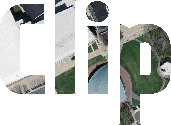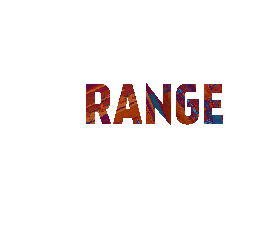Raster Words using PostGIS 2.1

Two of the big changes in PostGIS 2.1 raster are the improved speed and functionality of the raster ST_Union function and ST_Clip. Aside from speed, the big thing with ST_Union in 2.1 is that it applies operations to all bands by default. These are our most favorite funcitons of all. This is a continuation of Word Play with spatial SQL, except we'll be generating rasters instead of geometries and exercising some raster functions in addition to geometry functions.

Although these SQL statements look long and somewhat complicated, they are easily wrappable in an SQL function. We have, for example, an sql function to write letters on parcels that just takes as input the parcel id and the words to write.

This uses the postgis_letters extension, which we've finally put up on github postgis_letters. For rendering the images, we used our quickie viewer which relies on ASP.NET or PHP and JQuery. We'll be putting that up on github as well once we've dusted it off a bit.

If you are interested in the aerial and parcel geometry data we are using here, we grabbed it from MassGIS for Cambridge, Massachusetts area. You might recognize the base query in our upcoming DZone PostGIS refCard.

So here it goes. An exercise in raster expression.

Spatial SQLWord Image
``````WITH mit AS
(SELECT
-- union clipped resized tiles
ST_Union(
-- clip to 100 meter of parcel boundary
ST_Clip(
--resize to 25% of original
ST_Resize(a.rast,0.25,0.25)
,ST_Expand(p.geom,100))) AS rast
FROM aerials As a
INNER JOIN
parcels As p
-- select tiles within 100 meters of mit parcel
ON ST_DWithin(a.rast::geometry, p.geom, 100)
WHERE p.pid = '57-169E' )
, word AS (
SELECT  ST_LettersAsGeometry('Clip', 'kankin'
, ST_SRID(rast), 150, rast::geometry) As geom
FROM mit)
SELECT ST_Clip(mit.rast, word.geom ) As rast
FROM mit CROSS JOIN word;
````````````WITH mit AS
(SELECT ST_Union(ST_Clip(ST_Resize(a.rast,0.25,0.25)
,ST_Expand(p.geom,100))) AS rast
FROM aerials As a
INNER JOIN
parcels As p
ON ST_DWithin(a.rast::geometry, p.geom, 100)
WHERE p.pid = '57-169E' )
, word AS (SELECT
ST_AsRaster(ST_LettersAsGeometry('Mean', 'kankin', ST_SRID(rast)
, 50
-- start writing 50 meters (horizontally) before centroid
, ST_Translate(ST_Centroid(rast::geometry),-50,0) )
, rast
-- convert to 3 banded initialize 3 bands and make 0 no data value
, '{8BUI,8BUI,8BUI}'::text[]
, '{200,100,50}'::integer[]
, '{0,0,0}'::integer[]) As rast
FROM mit)
SELECT ST_Union(rast, 'MEAN') As rast
FROM (SELECT rast FROM mit
UNION ALL
SELECT rast
FROM word) AS final;
````````````WITH mit AS
(SELECT ST_Union(ST_Clip(ST_Resize(a.rast,0.25,0.25)
,ST_Expand(p.geom,100))) AS rast
FROM aerials As a
INNER JOIN
parcels As p
ON ST_DWithin(a.rast::geometry, p.geom, 100)
WHERE p.pid = '57-169E' )
, word AS (SELECT  ST_AsRaster(
ST_LettersAsGeometry('Last', 'kankin', ST_SRID(rast)
, 50
, ST_Translate(ST_Centroid(rast::geometry),-50,0) )
, rast
, '{8BUI,8BUI,8BUI}'::text[]
, '{200,100,50}'::integer[]
, '{0,0,0}'::integer[]) As rast
FROM mit)
SELECT ST_Union(rast, 'LAST') As rast
FROM (SELECT rast FROM mit
UNION ALL
SELECT rast
FROM word) AS final;
````````````WITH mit aS
(SELECT ST_Union(ST_Clip(ST_Resize(a.rast,0.25,0.25)
,ST_Expand(p.geom,100))) AS rast
FROM aerials As a
INNER JOIN
parcels As p
ON ST_DWithin(a.rast::geometry, p.geom, 100)
WHERE p.pid = '57-169E' )
, word AS (SELECT  ST_AsRaster(
ST_LettersAsGeometry('RANGE', 'kankin', ST_SRID(rast)
, 50
-- start writing 50 meters to left of centroid
, ST_Translate(ST_Centroid(rast::geometry),-50,0) )
, rast, '{8BUI,8BUI,8BUI}'::text[]
, '{200,100,50}'::integer[]
, '{0,0,0}'::integer[]) As rast
FROM mit)
SELECT ST_Union(rast, 'RANGE') As rast
FROM (SELECT rast FROM mit
UNION ALL
SELECT rast
FROM word) AS final;
`````````-- since SUM would exceed 255
-- when R band of RGB is added, R in area gets ceiling 255
-- this giving the sum a redish color
WITH mit aS
(SELECT ST_Union(ST_Clip(ST_Resize(a.rast,0.25,0.25)
,ST_Expand(p.geom,100))) AS rast
FROM aerials As a
INNER JOIN
parcels As p
ON ST_DWithin(a.rast::geometry, p.geom, 100)
WHERE p.pid = '57-169E' )
, word AS (SELECT  ST_AsRaster(ST_LettersAsGeometry('SUM', 'kankin', ST_SRID(rast)
, 50
-- start writing 50 meters to left of centroid
, ST_Translate(ST_Centroid(rast::geometry),-50,0) )
, rast, '{8BUI,8BUI,8BUI}'::text[], '{200,100,50}'::integer[], '{0,0,0}'::integer[]) As rast
FROM mit)
SELECT ST_Union(rast, 'SUM') As rast
FROM (SELECT rast FROM mit
UNION ALL
SELECT rast
FROM word) AS final;
```